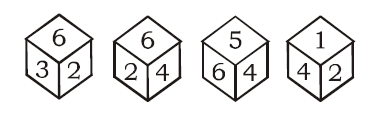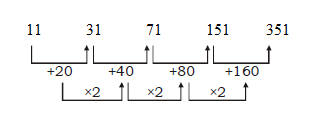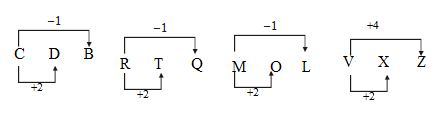# SSC CGL 2018 Practice Test Papers | Reasoning Ability (Day-21)

Dear Aspirants, Here we have given the Important SSC CGL Exam 2018 Practice Test Papers. Candidates those who are preparing for SSC CGL 2018 can practice these questions to get more confidence to Crack SSC CGL 2018 Examination.

[WpProQuiz 2972]

Click “Start Quiz” to attend these Questions and view Explanation

1) Nervous system: Neurology :: Lizards: ?

a) Phycology

b) Saurology

c) Pomology

d) Taxonomy

2) 11, 31, 71, 151, ?

a) 196

b) 256

c) 315

d) 351

3) Find the odd one.

a) Ears

b) Hands

c) Legs

d) Nose

4) Find the odd one.

a) CDB

b) RTQ

c) MOL

d) VXZ

5) Four positions of a dice are given below. Find the opposite surface of 6.a) 1

b) 3

c) 5

d) 4

6) In a certain code ‘2 6 3’ means ‘You and I’. ‘6 4 7’ means ‘You are bad’ and ‘7 5 2’ means ‘I am bad’. Then what is the code for ‘am’?

a) 3

b) 7

c) 4

d) 5

7) If ‘+’ means ‘–’, ‘–’ means ‘×’, ‘÷’ means ‘+’and ‘×’ means ‘÷’, then 20 × 10 ÷ 5 – 2 + 3 = ?

a) 21

b) 13

c) 17

d) 6

8) In a queue, the position of A from top is 7th and the position of B from top is 15th and21stfrom bottom. Now find the position of A from bottom?

a) 30

b) 15

c) 20

d) 29

9) A is brother of B, C is mother of A, D is father of C and F is A’s son. How is B related to F?

a) Uncle

b) Aunt

c) Father

d) Cannot be determined

10)  If 15 + 18 = 30, 25 + 32 = 24, 10 + 52 = 16 then, 14 + 21?

a) 15

b) 20

c) 26

d) 16

Study of nervous system is  Neurology.

Study of Lizards is SaurologyExcept d all others are 2 in number.From the diagram it is obvious that 2, 3, 4 and 5 are adjacent to 6. Therefore the face opposite to 6 is 1.

You – 6

I – 2

Am – 5

And – 3

Are – 4

(20 ÷ 10) + (5×2) – 3 = 17

10 + 10 – 3 = 17

Total = (Rank of B from top + Rank of B from bottom) – 1

= (15 + 21) – 1

= 35

Therefore A’s rank from bottom

=>35 = 7 + A – 1

= 35 = 6 + A

A = 35 – 6 = 29th

The gender of B is unknown. So it cannot be determined.

(1+5+1+8) x 2 = 30

(2+5+3+2) x 2 = 24

(1+0+5+2) x 2 = 16

(1+4+2+1) x 2 = 16